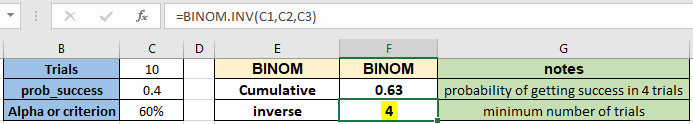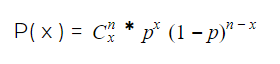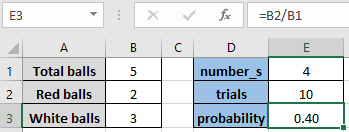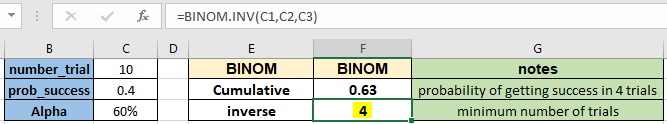# How to use the BINOM.INV function in ExcelIn this article, we will learn How to use the BINOM.INV function in Excel.

What is binomial probability distribution and inverse of binomial cumulative distribution?

The Binomial Distribution is a statistical measure that is mostly used to find the probability of a number of successes occurring from the number of independent trials. For instance, there are two outcomes of an event, either success expressed as p, or failure expressed 1-p. Probability p can take value in interval [0, 1]. The mathematical formula to find the expected value for binomial distribution of the event happening in x independent trials is shown below.Here C is the combinator function that can be translated as n! / (x! * (n-x)!) where ! is the symbol for factorial function.

The above formula is used to calculate the probability of an event happening in x independent trials. For the above function, you can use the BINOM.DIST function in Excel. Now taking the inverse of the above stated function we can calculate the smallest number of trials required for which the cumulative binomial distribution or critical value for the binomial distribution is greater than or equal to a criterion value or alpha. For example, the inverse of binomial cumulative distribution could be used to calculate the minimum number of tosses of a coin for which there is a 50% chance of at least 20 heads. Now you will understand how to calculate the smallest trial or event counts, the number of trials to have the first success using the BINOM.INV function.

BINOM.INV Function in Excel

BINOM.INV is a statistical function which returns the minimum value of trials for which the Cumulative Binomial Distribution function is a given probability.

BINOM.INV Function syntax:

 =BINOM.INV(trials, probability_s, alpha)

Trials : The number of independent trials.

Probability_s : The probability of success on each trial.

alpha : criterion value, the probability of the Cumulative Binomial Distribution (must be between 0 and 1).

Example :

All of these might be confusing to understand. Let's understand how to use the function using an example. Here we have a situation where we have 2 red balls and 3 white balls in a bag. We started an event where we take out a ball from the bag and note its color and put the ball back in the bag. If we repeat the task 10 times. For Now we want to know the smallest or minimum number of trials that could be required to get the red ball. So for the BINOM.INV function to be used we have a number of trials, probability successes and alpha probability. For that we use the basic formula of probability.

Use the formula:

 = number of red balls / total number of balls

Which will be interpreted as = B2 / B1.As you see, the probability of a red ball comes out to be 0.40. Using this probability value, we want the minimum number of trials required to get the red ball. Now we will use the BINOM.INV function with the given parameters.

Use the formula :

 =BINOM.INV( C1, C2, C3)As you can see, the minimum number of trials required are 4 which will definitely return the red ball or else check the bag again. whereas the probability to find the red ball in the next 4 trials or the binomial cumulative distribution comes out to be 0.63. You can use the function for any of the probability or cumulative functions. Use the BINOM.DIST function to calculate the probability value for the binomial distribution.

Here are all the observational notes using the BINOM.INV function in Excel
Notes :

1. The function only works with numbers.
2. Feed the arguments directly or using the cell reference as stated in the example above.
3. BINOM.INV function is the updated version of the CRITBINOM function.
4. trials are truncated to integers.
5. If alpha, trials, or probability_s is nonnumeric, BINOM.INV returns the #VALUE! error value.
6. If alpha < 0 or alpha > 1, BINOM.INV returns the #NUM! error value.
7. If probability_s < 0 or probability_s > 1, BINOM.INV returns the #NUM! error value.

Hope this article about How to use the BINOM.INV function in Excel is explanatory. Find more articles on statistical formulas and related Excel functions here. If you liked our blogs, share it with your friends on Facebook. And also you can follow us on Twitter and Facebook. We would love to hear from you, do let us know how we can improve, complement or innovate our work and make it better for you. Write to us at info@exceltip.com.

Related Articles :

How to use the VAR function in Excel : Calculate the variance for the sample dataset in excel using the VAR function in Excel.

How to Calculate Standard Deviation in Excel : To calculate the standard deviation we have different functions in Excel. The standard deviation is the square root of the variance value but It tells more about the dataset than variance.

Regressions Analysis in Excel : Regression is an Analysis Tool, which we use for analyzing large amounts of data and making forecasts and predictions in Microsoft Excel.

How to Create Standard Deviation Graph : The standard deviation tells how much data is clustered around the average of the data. Learn how to create a standard deviation graph here.

How to use Excel NORMDIST Function : Calculate the Z score for the normal cumulative distribution for the pre specified values using the NORMDIST function in Excel.

How to use Excel NORM.INV Function : Calculate the inverse of Z score for the normal cumulative distribution for the pre-specified probability values using the NORM.INV function in Excel.

Popular Articles :

How to use the IF Function in Excel : The IF statement in Excel checks the condition and returns a specific value if the condition is TRUE or returns another specific value if FALSE.

How to use the VLOOKUP Function in Excel : This is one of the most used and popular functions of excel that is used to lookup value from different ranges and sheets.

How to Use SUMIF Function in Excel : This is another dashboard essential function. This helps you sum up values on specific conditions.

How to use the COUNTIF Function in Excel : Count values with conditions using this amazing function. You don't need to filter your data to count specific values. Countif function is essential to prepare your dashboard.

Terms and Conditions of use

The applications/code on this site are distributed as is and without warranties or liability. In no event shall the owner of the copyrights, or the authors of the applications/code be liable for any loss of profit, any problems or any damage resulting from the use or evaluation of the applications/code.